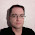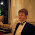## Tuesday, December 12, 2017

### More about GCC instruction patterns

This is part five of a series “Writing a GCC back end”.

The previous post about GCC’s low-level IR did only contain the minimum to get started – this post continues with a bit more of the functionality used in machine descriptions.

### define_expand

The define_insn definition describes an insn implementing the named functionality (used when converting the higher level IR to RTL), but there are cases where the named functionality must expand to more than one insn, or when the RTL should be generated differently depending on the operands – this is handled by define_expand.

The general format of define_expand is essentially the same as for define_insn – it consists of a name, an RTL template, a condition string containing C++ code to enable/disable the instruction pattern, and a section of C++ code (called preparation statements) that can be used to generate RTL.

Our first example of how to use define_expand is from the Arm handling of rotlsi3 (“rotate left”) – Arm does only have a “rotate right” instruction, but rotating left by x is the same as rotating right by 32-x, and we can generate this as:
(define_expand "rotlsi3"
[(set (match_operand:SI              0 "s_register_operand" "")
(rotatert:SI (match_operand:SI 1 "s_register_operand" "")
(match_operand:SI 2 "reg_or_int_operand" "")))]
"TARGET_32BIT"
{
if (CONST_INT_P (operands))
operands = GEN_INT ((32 - INTVAL (operands)) % 32);
else
{
rtx reg = gen_reg_rtx (SImode);
emit_insn (gen_subsi3 (reg, GEN_INT (32), operands));
operands = reg;
}
})

The preparation statements are used to modify the second operand given to rotlsi3 to calculate the correct value for the code generated by the RTL template.

define_expand may choose to skip the generation of the RTL template by executing DONE in the preparation statements. An example of how this is used is the Moxie implementation of mulsidi3 that creates a 64-bit result from multiplying two 32-bit values. The Moxie back end generates this pattern as two instructions (one that calculates the upper 32 bits, and one that calculates the lower 32 bits) where all work is done by the preparation statements
(define_expand "mulsidi3"
[(set (match_operand:DI 0 "register_operand" "")
(mult:DI (sign_extend:DI (match_operand:SI 1 "register_operand" "0"))
(sign_extend:DI (match_operand:SI 2 "register_operand" "r"))))]
""
{
rtx hi = gen_reg_rtx (SImode);
rtx lo = gen_reg_rtx (SImode);

emit_insn (gen_mulsi3_highpart (hi, operands, operands));
emit_insn (gen_mulsi3 (lo, operands, operands));
emit_move_insn (gen_lowpart (SImode, operands), lo);
emit_move_insn (gen_highpart (SImode, operands), hi);
DONE;
})

Note: This define_expand contains an RTL template, but it is not needed as RTL will not be generated from it. It would have been enough to specify the operands, as in
(define_expand "mulsidi3"
[(match_operand:DI 0 "register_operand")
(match_operand:SI 1 "register_operand")
(match_operand:SI 2 "register_operand")]
""
{
rtx hi = gen_reg_rtx (SImode);
rtx lo = gen_reg_rtx (SImode);

emit_insn (gen_mulsi3_highpart (hi, operands, operands));
emit_insn (gen_mulsi3 (lo, operands, operands));
emit_move_insn (gen_lowpart (SImode, operands), lo);
emit_move_insn (gen_highpart (SImode, operands), hi);
DONE;
})


DONE works as a return statement, and it can be used in conditional code to let the preparation statements override the RTL template for special cases while using the RTL template for the general case. The Arm smaxsi3 instruction pattern (calculating the maximum of two signed integers) uses this to handle two special cases more efficiently:
(define_expand "smaxsi3"
[(parallel [
(set (match_operand:SI 0 "s_register_operand" "")
(smax:SI (match_operand:SI 1 "s_register_operand" "")
(match_operand:SI 2 "arm_rhs_operand" "")))
(clobber (reg:CC CC_REGNUM))])]
"TARGET_32BIT"
{
if (operands == const0_rtx || operands == constm1_rtx)
{
/* No need for a clobber of the condition code register here.  */
emit_insn (gen_rtx_SET (operands,
gen_rtx_SMAX (SImode, operands,
operands)));
DONE;
}
})

The problem it solves is that the general case translates to the instructions
cmp   r1, r2
movge r0, r2
movlt r0, r1

which clobbers the condition code, but the special cases max(x,0) and max(x,-1) can be generated as
bic   r0, r1, r1, asr #31

and
orr   r0, r1, r1, asr #31

which do not clobber CC. This optimization could be handled by later optimization passes, but doing it as early as possible (i.e. when generating the RTL) gives more freedom to all later passes to optimize cases that otherwise would have been prevented by the clobbering.

Note: The RTL always have the constant as the second operand for commutative binary operations, so the code does not need to check the first operand.

One other way to return from the preparation statements is to execute FAIL which has the effect of ignoring the instruction pattern in the same way as if the pattern had been disabled by the condition string. An example of this is the AArch64 movmemdi instruction pattern implementing a memory block move:
(define_expand "movmemdi"
[(match_operand:BLK 0 "memory_operand")
(match_operand:BLK 1 "memory_operand")
(match_operand:DI 2 "immediate_operand")
(match_operand:DI 3 "immediate_operand")]
"!STRICT_ALIGNMENT"
{
if (aarch64_expand_movmem (operands))
DONE;
FAIL;
})

This calls the target-specific aarch64_expand_movmem function that checks if it makes sense to expand the block move inline (that is, if the result will be relatively small) and generates a sequence of move insns if that is the case. If not, it just returns false, which makes this pattern call FAIL, and GCC will ignore this instruction pattern and generate a call to memcpy instead.

### The unspec and unspec_volatile expression codes

The RTL template in define_insn contains expressions describing the functionality of the instruction pattern, which enables the optimizers to reason about the insn. Many architectures have instructions that cannot be described by this (or where it does not make sense to describe the functionality, such as instructions for AES encryption – the optimizers cannot take advantage of this description anyway). These cases are handled by describing the instructions using an unspec or unspec_volatile expression which the compiler treats as a black box – the only knowledge the compiler has is what is described by the predicates and register constraints.

One example of how this is used the AArch64 set_fpsr insn that writes to the floating-point status register
(define_insn "set_fpsr"
[(unspec_volatile [(match_operand:SI 0 "register_operand" "r")] UNSPECV_SET_FPSR)]
""
"msr\\tfpsr, %0")

This describes a volatile instruction (i.e. an instruction with side effects) that takes a register operand. The last operand to the unspec and unspec_volatile expressions is an integer that identifies the instruction (the backend may have several different unspec instructions, and each gets a different number) – these are by convention defined as enumerations called unspec and unspecv
(define_c_enum "unspecv" [
UNSPECV_EH_RETURN           ; Represent EH_RETURN
UNSPECV_GET_FPCR            ; Represent fetch of FPCR content.
UNSPECV_SET_FPCR            ; Represent assign of FPCR content.
UNSPECV_GET_FPSR            ; Represent fetch of FPSR content.
UNSPECV_SET_FPSR            ; Represent assign of FPSR content.
UNSPECV_BLOCKAGE            ; Represent a blockage
UNSPECV_PROBE_STACK_RANGE   ; Represent stack range probing.
])


### Attributes

It is possible to add extra information to an insn (such as the length or scheduling constraints) that the back end may take advantage of when generating the code. This is done by defining attributes
(define_attr name list-of-values default)

where
• name is a string containing the name of the attribute.
• list-of-values is either a string that specifies a comma-separated list of values that can be assigned to the attribute, or an empty string to specify that the attribute takes numeric values.
• default is an expression that gives the value of this attribute for insns whose definition does not include an explicit value for the attribute.
For example,
(define_attr "length" "" (const_int 2))

defines a numeric attribute “length” having the default value 2. The back end may define attributes with any name, but a few names have a specific usage in GCC. For example, the length attribute contains the instruction’s length measuerd in bytes, and is used when calculating branch distance for architectures where different instruction are used for “short” and “long” branches.

Attribute values are assigned to insns by attaching a set_attr to the define_insn as in
(define_insn "*call"
[(call (mem:QI (match_operand:SI 0 "nonmemory_operand" "i,r"))
(match_operand 1 "" ""))]
""
"@
jsra\\t%0
jsr\\t%0"
[(set_attr "length" "6,2")])

This gives the length attribute the value 6 if the first alternative was matched, and 2 if the second alternative was matched.

The attributes can be accessed from C++ code by calling the auto-generated function get_attr_name, as in
int len = get_attr_length (insn);

The return type of get_attr_name for attributes defined with a list-of-values is an enum of the possible values.

All functionality is described in “GNU Compiler Collection Internals”:

1.This is great. How are the unspec instructions like set_fpsr used?

1.The $$\verb!unspec!$$ expression is not different from the usual expressions such as $$\verb!mult:SI!$$ – you could have defined the $$\verb!mulsi3!$$ insn from the previous blog post using an $$\verb!unspec!$$ expression, and GCC would generate it for multiplications in exactly the same way (but it would not be able to optimize as much, as the back end will not understand what the operation is doing...).

So you create the $$\verb!set_fpsr!$$ by calling $$\verb!emit_insn!$$ as usual (typically from an $$\verb!define_expand!$$).

2.Thanks, it makes sense now. Also I found that during compilation the unspec instructions generate a helper function in gcc/insn-emit.c; in this case gen_set_fpsr. I guess that is true for all instructions, but I didn't realise it.

2.I am trying to modify gcc backed for RISC-V.
I am using define_expand to expand "mul3" functionality to two RTL insns:

1. modifiedfmul: this RTL insn is same as the predefined mul3 instruction which i am trying to modify.

2. riscv_frflags

The build fails with the message:
../../../riscv-gcc/gcc/config/riscv/riscv.md:578:1: duplicate definition of 'modifiedfmul'.

1.It is hard to tell from your description, but I’m happy to take a look at the problem if you send me a patch with your changes (krister.walfridsson at gmail.com).

2.Thanks Krister for the reply. I works fine now.
The problem was due to the use of iterator INF in 'modifiedfmul'. The iterator was creating multiple patterns, for each mode.